nextnano.com  GUI: nextnanomat  Tool: nextnano++  Tool: nextnano³  Tool: nextnano.QCL  Download | Search | Copyright | News | Publications  * password protected nextnano³ software1D Poisson

# nextnano3 - Tutorial

## Solution of the Poisson equation for different charge density profiles

Author: Stefan Birner

If you want to obtain the input files that are used within this tutorial, please contact stefan.birner@nextnano.de.
`1) -> 1D_Poisson_dipole.in / *_nnp.in - `input file for the nextnano3 and nextnano++ software (1D simulation)``` 2) -> 1D_Poisson_linear.in 3) -> 1D_Poisson_delta.in  ```

## 1) Dipole: Constant charge density profile of positive and negative charge

The following figures show a dipole charge density distribution where
`- `the left   region carries a constant positive  charge density (resulting from ionized donors     ND+) and
`- `the right region carries a constant negative charge density (resulting from ionized acceptors NA`-`).

Left figure:`  1Ddoping_concentration.dat`
Right figure:` densities/density1Dspace_charge.dat`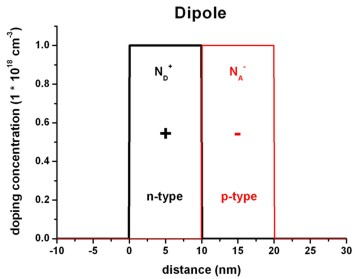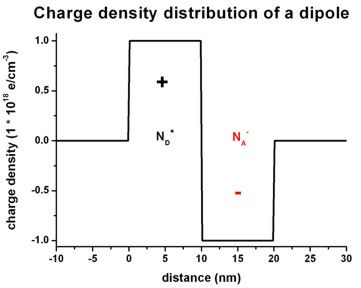We have to solve the Poisson equation: d2phi / dx2 = `-` rho / (epsilon epsilon0)

The following figures shows the corresponding electric field distribution (left) and the electrostatic potential (right).

Left figure:`  band_structure/electric_field1D.dat`
Right figure:` band_structure/potential1D.dat`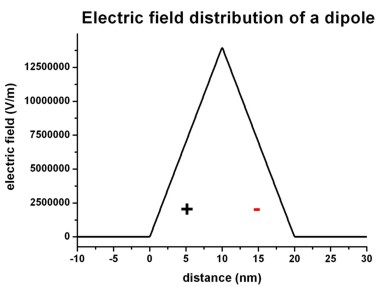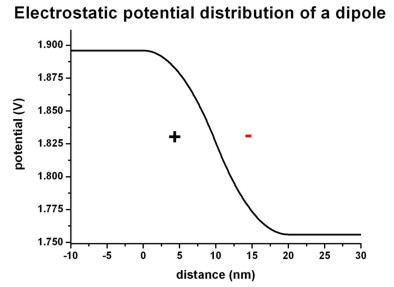The electric field is given by E(x) = `-` dphi / dx and has a linear dependence (~ `-`x) because the electrostatic potential has a quadratic dependence (~ x2).

The maximum value of the electric field is given by:

Emax = rho / (epsilon epsilon0) * x0 = e * 1*1018 cm`-`3 / ( 12.93 * 8.8542*10`-`12 As/Vm ) * 10 nm =
= 1.3995*107 V/m = 139.95 kV/cm``` ```where x0 is the width of the positive (or negative) charge density region, and epsilon = 12.93 is the static dielectric constant of GaAs.

The drop of the electrostatic potential between 0 nm and 20 nm is simply given by the area that is below the graph of the electric field:

Delta phi = 1/2 Emax * 20 nm = 139.95 mV

## 2) Linear charge density profile of positive and negative charge

The following figures show a linearly varying charge density distribution where
`- `the left   region carries a linearly decreasing positive  charge density (resulting from ionized donors     ND+) and
`- `the right region carries a linearly increasing  negative charge density (resulting from ionized acceptors NA`-`).

Left figure:`  1Ddoping_concentration.dat`
Right figure:` densities/density1Dspace_charge.dat`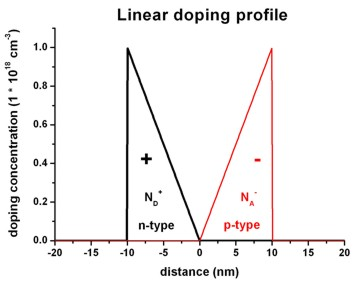The following figures shows the corresponding electric field distribution (left) and the electrostatic potential (right).

Left figure:`  band_structure/electric_field1D.dat`
Right figure:` band_structure/potential1D.dat`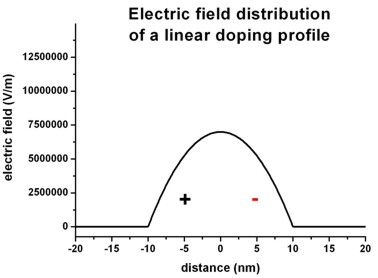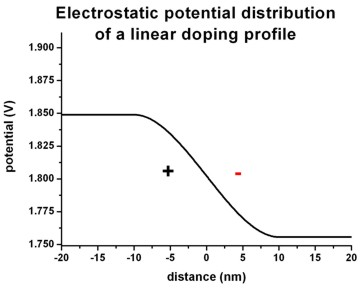The electric field shows a quadratic dependence  (~ `-`x2) whereas the electrostatic potential shows a cubic dependence (~ x3).

## 3) Delta-function like charge density profile of positive and negative charges

The following figures show a delta-function like charge density distribution where
`- `in the middle       of the structure there is a constant positive  charge density   of width 1 nm         (resulting from ionized donors     ND+) and
`- `at the boundaries of the structure there are constant negative charge densities of width 1 nm each (resulting from ionized acceptors NA`-`).

Left figure:`  1Ddoping_concentration.dat`
Right figure:` densities/density1Dspace_charge.dat`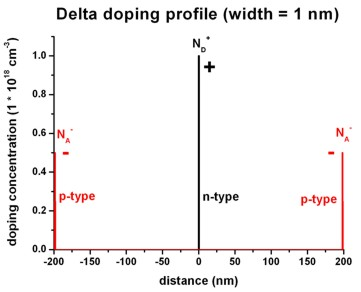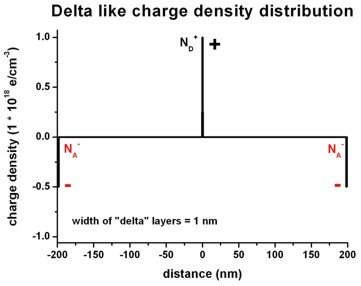The following figures shows the corresponding electric field distribution (left) and the electrostatic potential (right).

Left figure:`  band_structure/electric_field1D.dat`
Right figure:` band_structure/potential1D.dat`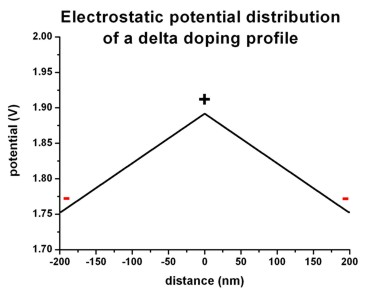• Please help us to improve our tutorial. Send comments to``` support [at] nextnano.com```.